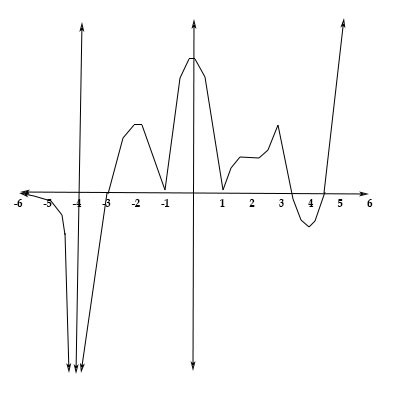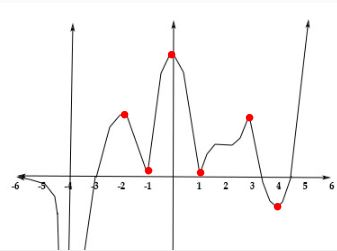# Use the given graph of f(x) to determine the intervals where f'(x) >0, f'(x) ,0 and the x values...

## Question:

Use the given graph of {eq}f(x) {/eq} to determine the intervals where {eq}f'(x) >0, f'(x) ,0 {/eq} and the {eq}x {/eq} values where {eq}f'(x) = 0, {/eq} and where {eq}f'(x) {/eq} is undefined.

Then sketch a graph of {eq}f'(x). {/eq}## Critical Points:

We can find the critical points of a function by looking at the graph of the function, the critical points are where the curve ends to increase and begins to decrease and when the curve ends to decrease and begins to increase.

We have the functionWe can find the intervals that are increasing {eq}f' >0 \\ {/eq} at {eq}(-4, -2] \\ [-1, 0] \\ [1, 3] \\ [4, \infty ) \\ {/eq}

When the first derivative of the function is zero we can find the critical points of the function. in this case:

{eq}f'(x)=0 {/eq} when {eq}x= -2 \\ x=-1 \\ x=0 \\ x=1 \\ x=3 \\ x=4 \\ {/eq}

The first derivative is undefined in the asymptotes that the function has. In this case, the function has a vertical asymptote. Therefore:

{eq}f'(x) {/eq} is undefined at {eq}x=-4 \\ {/eq}